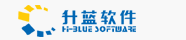# 用SQL Server为Web浏览器提供图像（四）(转)

March 25,2004

</OBJECT>
<SCRIPT LANGUAGE=VBScript RUNAT=Server>
Sub Session_OnStart
Session("Grocevb_ConnectionString") = "DRIVER=SQL Server; SERVER=(local);
UID=sa;PWD=;APP=Microsoft (R) Developer Studio;WSID=JIA;
DATABASE=Grocevb;UseProcForPrepare=0"
Session("Grocevb_ConnectionTimeout") = 15
Session("Grocevb_CommandTimeout") = 30
End Sub

Sub Session_OnEnd
End Sub

</SCRIPT>

dbConn.ConnectionTimeout = Session("Grocevb_ConnectionTimeout")
dbConn.CommandTimeout = Session("Grocevb_CommandTimeout")

cmdTemp.CommandText = "SELECT prod_id, prod_name, prod_desc, prod_unitprice, & _
" prod_unitquant, prod_imagetype " & _
"FROM Products ORDER BY prod_name"
cmdTemp.CommandType = 1
Set cmdTemp.ActiveConnection = dbConn
rstProduct.Open cmdTemp, , 0, 1

%>

<HTML>
<TITLE>从SQL Server装载图片的示例</TITLE>
<BODY bgcolor="#FFFFFF" TOPMARGIN=8 LEFTMARGIN=4>
<%Do While Not rstProduct.EOF %>
<%ProdId = rstProduct("prod_id") %>
<% FileName =  "images/" & objImageLoader.ImageFile(ProdId) %>

<TABLE border=0 cellpadding=5 cellspacing=0 width=100% cols=2>
<td width=30%>
<IMG ALIGN=BOTTOM SRC= <% =FileName %>>
<p> </p>
<font size=2>Image type: <% = rstProduct("prod_imagetype") %>
</td>
<td width=70%>
<p align=center><font size=4><% =rstProduct("prod_name") %></font></p>
<p align=center><% =rstProduct("prod_desc") %></p>
</td>
</TABLE>
<p> </p>
<TABLE border=0 cellpadding=5, cellspacing=0 width=100% cols=2>
<td width=50%>
<p align=left>Unit quantity: <% = rstProduct("prod_unitquant") %></P>
</td>
<td width=50%>
<p align=left>Unit price: <% =rstProduct("prod_unitprice") %></p>
</td>
</TABLE>
</TABLE>
<p> </p>
<% rstProduct.MoveNext %>
<% Loop %>
</BODY>
</HTML>